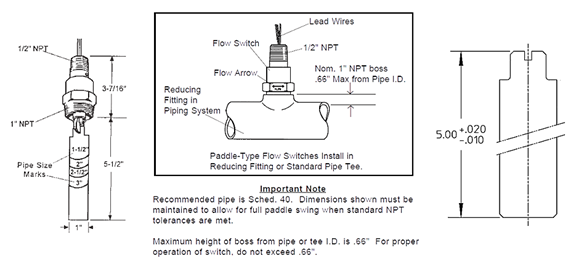## FS-550 Series Long Paddle Switch Actuation in Pipe Sizes Over 5"

The FS-550 long paddle is 5" uncut measured from cam pivot pin. Always consider all dimensions when installing.To determine the GPM switch actuation point for larger pipes over 5” with an uncut paddle, use the following guide and equation:

Q = VA

Where Q is flow rate in GPM, V is fluid velocity in ft/sec, and A is inside diameter pipe area in sq in.

For calculations of flow rates (Q) in pipe sizes larger than 5”, a flow velocity (V) of approximately 0.5 ft per sec actuates the full length (5”) paddle.

• So, V =0.5

Inner pipe area (A) is calculated by measuring the pipe diameter, multiplying that by itself (squared, D2), then multiplying that value by Pi (3.14), then divide that value by 4.

• So, A = D2 * 3.14 ÷ 4

When calculating your final result, to solve for Q you need to use the 1 cu ft/sec = 448.8312 GPM velocity conversion then convert from cubic feet to square inches (÷144).

• So, Q = 0.5 * A ÷ 144 * 448.8312

Here's a practical example of std schedule 40 pipe size of 6":

• V = 0.5
• A = 28.26 (62 * 3.14 ÷ 4)
• Q = 0.5 * 28.26 ÷ 144 * 448.8312
• Q = 44.04156 GPM

Your 6” diameter pipe with uncut long paddle FS-550 will have appx 44 GPM actuation switch point.

Please note, the setpoint accuracy is ± 25% of GPM setpoint. Also note de-actuation point will fall somewhere below actuation point (appx ≥ 14GPM less).

FS-550 Reference:

Landing Page

Catalog Page

Instruction Bulletin